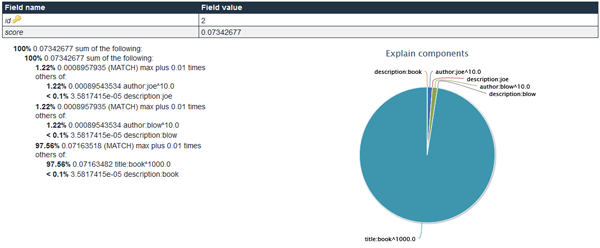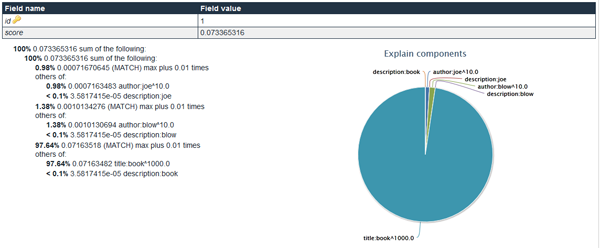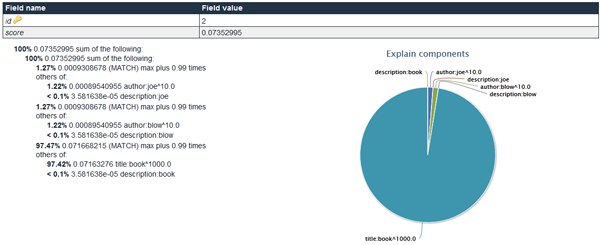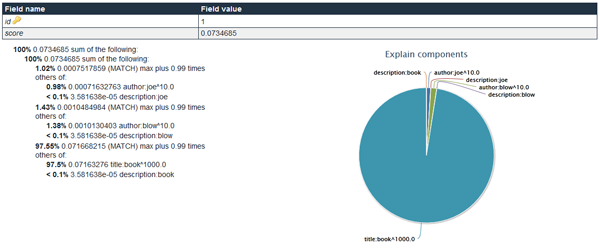# What can we use Dismax tie parameter for?

Dismax query parser have been with Solr for a long time. Most of the time we use parameters like qf, pf or mm forgetting about a very useful parameter which allows us to control how the lower scoring fields are treated – about the tie parameter.

### Tie

The tie parameter allows one to control how the lower scoring fields affects score for a given word. If we set the tie parameter to a 0.0 value, during the score calculation, only the fields that were scored highest will matter. However if we set it to 0.99 the fields scoring lower will have almost the same impact on the score as the highest scoring field. So let’s check if that actually works.

### Data structure and data example

To test how the tie parameter works I’ve chosen a simple index structure which would describe products in e-commerce shop, of course in a simple mode:

```<field name="id" type="string" indexed="true" stored="true" required="true" />
<field name="title" type="text_ws" indexed="true" stored="true" />
<field name="description" type="text_ws" indexed="true" stored="true" />
<field name="author" type="text_ws" indexed="true" stored="true" multiValued="true" />```

The text_ws type was defined as follows:

```<fieldType name="text_ws" class="solr.TextField" positionIncrementGap="100">
<analyzer>
<tokenizer class="solr.WhitespaceTokenizerFactory"/>
<filter class="solr.LowerCaseFilterFactory"/>
</analyzer>
</fieldType>```

The example documents look like this:

```<add>
<doc>
<field name="id">1</field>
<field name="title">First test book</field>
<field name="description">This is a description of the first test book by Joe and Jane Blow</field>
<field name="author">Joe Blow</field>
<field name="author">Jane Blow</field>
</doc>
<doc>
<field name="id">2</field>
<field name="title">Second test book</field>
<field name="description">This is a description of the second test book by Joe Blow</field>
<field name="author">Joe Blow</field>
</doc>

### Tie == 0.01 result

Let’s start the test. The first query was the following one:

`defType=dismax&qf=title^1000 description author^10&tie=0.01&fl=id,score&debugQuery=on&indent=true&q=joe blow book`

The above resulted in the following Solr results (visualization – http://explain.solr.pl/explains/cf0wnkpj):

```<?xml version="1.0" encoding="UTF-8"?>
<response>
<int name="status">0</int>
<int name="QTime">8</int>
<lst name="params">
<str name="fl">id,score</str>
<str name="debugQuery">on</str>
<str name="indent">true</str>
<str name="tie">0.01</str>
<str name="q">joe blow book</str>
<str name="qf">title^1000 description author^10</str>
<str name="defType">dismax</str>
</lst>
</lst>
<result name="response" numFound="2" start="0" maxScore="0.07342677">
<doc>
<float name="score">0.07342677</float>
<str name="id">2</str>
</doc>
<doc>
<float name="score">0.073365316</float>
<str name="id">1</str>
</doc>
</result>
<lst name="debug">
<str name="rawquerystring">joe blow book</str>
<str name="querystring">joe blow book</str>
<str name="parsedquery">+((DisjunctionMaxQuery((author:joe^10.0 | title:joe^1000.0 | description:joe)~0.01) DisjunctionMaxQuery((author:blow^10.0 | title:blow^1000.0 | description:blow)~0.01) DisjunctionMaxQuery((author:book^10.0 | title:book^1000.0 | description:book)~0.01))~3) ()</str>
<str name="parsedquery_toString">+(((author:joe^10.0 | title:joe^1000.0 | description:joe)~0.01 (author:blow^10.0 | title:blow^1000.0 | description:blow)~0.01 (author:book^10.0 | title:book^1000.0 | description:book)~0.01)~3) ()</str>
<lst name="explain">
<str name="2">
0.07342677 = (MATCH) sum of:
0.07342677 = (MATCH) sum of:
8.957935E-4 = (MATCH) max plus 0.01 times others of:
8.9543534E-4 = (MATCH) weight(author:joe^10.0 in 1), product of:
0.0024097771 = queryWeight(author:joe^10.0), product of:
10.0 = boost
0.5945349 = idf(docFreq=2, maxDocs=2)
4.0532142E-4 = queryNorm
0.3715843 = (MATCH) fieldWeight(author:joe in 1), product of:
1.0 = tf(termFreq(author:joe)=1)
0.5945349 = idf(docFreq=2, maxDocs=2)
0.625 = fieldNorm(field=author, doc=1)
3.5817415E-5 = (MATCH) weight(description:joe in 1), product of:
2.4097772E-4 = queryWeight(description:joe), product of:
0.5945349 = idf(docFreq=2, maxDocs=2)
4.0532142E-4 = queryNorm
0.14863372 = (MATCH) fieldWeight(description:joe in 1), product of:
1.0 = tf(termFreq(description:joe)=1)
0.5945349 = idf(docFreq=2, maxDocs=2)
0.25 = fieldNorm(field=description, doc=1)
8.957935E-4 = (MATCH) max plus 0.01 times others of:
8.9543534E-4 = (MATCH) weight(author:blow^10.0 in 1), product of:
0.0024097771 = queryWeight(author:blow^10.0), product of:
10.0 = boost
0.5945349 = idf(docFreq=2, maxDocs=2)
4.0532142E-4 = queryNorm
0.3715843 = (MATCH) fieldWeight(author:blow in 1), product of:
1.0 = tf(termFreq(author:blow)=1)
0.5945349 = idf(docFreq=2, maxDocs=2)
0.625 = fieldNorm(field=author, doc=1)
3.5817415E-5 = (MATCH) weight(description:blow in 1), product of:
2.4097772E-4 = queryWeight(description:blow), product of:
0.5945349 = idf(docFreq=2, maxDocs=2)
4.0532142E-4 = queryNorm
0.14863372 = (MATCH) fieldWeight(description:blow in 1), product of:
1.0 = tf(termFreq(description:blow)=1)
0.5945349 = idf(docFreq=2, maxDocs=2)
0.25 = fieldNorm(field=description, doc=1)
0.07163518 = (MATCH) max plus 0.01 times others of:
0.07163482 = (MATCH) weight(title:book^1000.0 in 1), product of:
0.2409777 = queryWeight(title:book^1000.0), product of:
1000.0 = boost
0.5945349 = idf(docFreq=2, maxDocs=2)
4.0532142E-4 = queryNorm
0.29726744 = (MATCH) fieldWeight(title:book in 1), product of:
1.0 = tf(termFreq(title:book)=1)
0.5945349 = idf(docFreq=2, maxDocs=2)
0.5 = fieldNorm(field=title, doc=1)
3.5817415E-5 = (MATCH) weight(description:book in 1), product of:
2.4097772E-4 = queryWeight(description:book), product of:
0.5945349 = idf(docFreq=2, maxDocs=2)
4.0532142E-4 = queryNorm
0.14863372 = (MATCH) fieldWeight(description:book in 1), product of:
1.0 = tf(termFreq(description:book)=1)
0.5945349 = idf(docFreq=2, maxDocs=2)
0.25 = fieldNorm(field=description, doc=1)
</str>
<str name="1">
0.073365316 = (MATCH) sum of:
0.073365316 = (MATCH) sum of:
7.1670645E-4 = (MATCH) max plus 0.01 times others of:
7.163483E-4 = (MATCH) weight(author:joe^10.0 in 0), product of:
0.0024097771 = queryWeight(author:joe^10.0), product of:
10.0 = boost
0.5945349 = idf(docFreq=2, maxDocs=2)
4.0532142E-4 = queryNorm
0.29726744 = (MATCH) fieldWeight(author:joe in 0), product of:
1.0 = tf(termFreq(author:joe)=1)
0.5945349 = idf(docFreq=2, maxDocs=2)
0.5 = fieldNorm(field=author, doc=0)
3.5817415E-5 = (MATCH) weight(description:joe in 0), product of:
2.4097772E-4 = queryWeight(description:joe), product of:
0.5945349 = idf(docFreq=2, maxDocs=2)
4.0532142E-4 = queryNorm
0.14863372 = (MATCH) fieldWeight(description:joe in 0), product of:
1.0 = tf(termFreq(description:joe)=1)
0.5945349 = idf(docFreq=2, maxDocs=2)
0.25 = fieldNorm(field=description, doc=0)
0.0010134276 = (MATCH) max plus 0.01 times others of:
0.0010130694 = (MATCH) weight(author:blow^10.0 in 0), product of:
0.0024097771 = queryWeight(author:blow^10.0), product of:
10.0 = boost
0.5945349 = idf(docFreq=2, maxDocs=2)
4.0532142E-4 = queryNorm
0.42039964 = (MATCH) fieldWeight(author:blow in 0), product of:
1.4142135 = tf(termFreq(author:blow)=2)
0.5945349 = idf(docFreq=2, maxDocs=2)
0.5 = fieldNorm(field=author, doc=0)
3.5817415E-5 = (MATCH) weight(description:blow in 0), product of:
2.4097772E-4 = queryWeight(description:blow), product of:
0.5945349 = idf(docFreq=2, maxDocs=2)
4.0532142E-4 = queryNorm
0.14863372 = (MATCH) fieldWeight(description:blow in 0), product of:
1.0 = tf(termFreq(description:blow)=1)
0.5945349 = idf(docFreq=2, maxDocs=2)
0.25 = fieldNorm(field=description, doc=0)
0.07163518 = (MATCH) max plus 0.01 times others of:
0.07163482 = (MATCH) weight(title:book^1000.0 in 0), product of:
0.2409777 = queryWeight(title:book^1000.0), product of:
1000.0 = boost
0.5945349 = idf(docFreq=2, maxDocs=2)
4.0532142E-4 = queryNorm
0.29726744 = (MATCH) fieldWeight(title:book in 0), product of:
1.0 = tf(termFreq(title:book)=1)
0.5945349 = idf(docFreq=2, maxDocs=2)
0.5 = fieldNorm(field=title, doc=0)
3.5817415E-5 = (MATCH) weight(description:book in 0), product of:
2.4097772E-4 = queryWeight(description:book), product of:
0.5945349 = idf(docFreq=2, maxDocs=2)
4.0532142E-4 = queryNorm
0.14863372 = (MATCH) fieldWeight(description:book in 0), product of:
1.0 = tf(termFreq(description:book)=1)
0.5945349 = idf(docFreq=2, maxDocs=2)
0.25 = fieldNorm(field=description, doc=0)
</str>
</lst>
</lst>
</response>```

#### First document#### Second document#### What can we say about that ?

As you can see, when we passed the 0.01 value to the tie parameter, only those fields that have the highest score for the given query word are most influential. Good example of that behavior is the book word in the first document on the results list. Score for that word is `0.07163518`, which was calculated as the sum of the highest scored field (the title field) and the sum of the rest of the fields multiplied by tie.

### Tie == 0.99 result

The second query sent to Solr looked as follows:

`defType=dismax&qf=title^1000 description author^10&tie=0.99&fl=id,score&debugQuery=on&indent=true&q=joe blow book`

Which resulted in the following Solr results: (visualization – http://explain.solr.pl/explains/1w7b06lv):

```<?xml version="1.0" encoding="UTF-8"?>
<response>
<int name="status">0</int>
<int name="QTime">15</int>
<lst name="params">
<str name="fl">id,score</str>
<str name="debugQuery">on</str>
<str name="indent">true</str>
<str name="tie">0.99</str>
<str name="q">joe blow book</str>
<str name="qf">title^1000 description author^10</str>
<str name="defType">dismax</str>
</lst>
</lst>
<result name="response" numFound="2" start="0" maxScore="0.07352995">
<doc>
<float name="score">0.07352995</float>
<str name="id">2</str>
</doc>
<doc>
<float name="score">0.0734685</float>
<str name="id">1</str>
</doc>
</result>
<lst name="debug">
<str name="rawquerystring">joe blow book</str>
<str name="querystring">joe blow book</str>
<str name="parsedquery">+((DisjunctionMaxQuery((author:joe^10.0 | title:joe^1000.0 | description:joe)~0.99) DisjunctionMaxQuery((author:blow^10.0 | title:blow^1000.0 | description:blow)~0.99) DisjunctionMaxQuery((author:book^10.0 | title:book^1000.0 | description:book)~0.99))~3) ()</str>
<str name="parsedquery_toString">+(((author:joe^10.0 | title:joe^1000.0 | description:joe)~0.99 (author:blow^10.0 | title:blow^1000.0 | description:blow)~0.99 (author:book^10.0 | title:book^1000.0 | description:book)~0.99)~3) ()</str>
<lst name="explain">
<str name="2">
0.07352995 = (MATCH) sum of:
0.07352995 = (MATCH) sum of:
9.308678E-4 = (MATCH) max plus 0.99 times others of:
8.9540955E-4 = (MATCH) weight(author:joe^10.0 in 1), product of:
0.0024097078 = queryWeight(author:joe^10.0), product of:
10.0 = boost
0.5945349 = idf(docFreq=2, maxDocs=2)
4.0530972E-4 = queryNorm
0.3715843 = (MATCH) fieldWeight(author:joe in 1), product of:
1.0 = tf(termFreq(author:joe)=1)
0.5945349 = idf(docFreq=2, maxDocs=2)
0.625 = fieldNorm(field=author, doc=1)
3.581638E-5 = (MATCH) weight(description:joe in 1), product of:
2.4097077E-4 = queryWeight(description:joe), product of:
0.5945349 = idf(docFreq=2, maxDocs=2)
4.0530972E-4 = queryNorm
0.14863372 = (MATCH) fieldWeight(description:joe in 1), product of:
1.0 = tf(termFreq(description:joe)=1)
0.5945349 = idf(docFreq=2, maxDocs=2)
0.25 = fieldNorm(field=description, doc=1)
9.308678E-4 = (MATCH) max plus 0.99 times others of:
8.9540955E-4 = (MATCH) weight(author:blow^10.0 in 1), product of:
0.0024097078 = queryWeight(author:blow^10.0), product of:
10.0 = boost
0.5945349 = idf(docFreq=2, maxDocs=2)
4.0530972E-4 = queryNorm
0.3715843 = (MATCH) fieldWeight(author:blow in 1), product of:
1.0 = tf(termFreq(author:blow)=1)
0.5945349 = idf(docFreq=2, maxDocs=2)
0.625 = fieldNorm(field=author, doc=1)
3.581638E-5 = (MATCH) weight(description:blow in 1), product of:
2.4097077E-4 = queryWeight(description:blow), product of:
0.5945349 = idf(docFreq=2, maxDocs=2)
4.0530972E-4 = queryNorm
0.14863372 = (MATCH) fieldWeight(description:blow in 1), product of:
1.0 = tf(termFreq(description:blow)=1)
0.5945349 = idf(docFreq=2, maxDocs=2)
0.25 = fieldNorm(field=description, doc=1)
0.071668215 = (MATCH) max plus 0.99 times others of:
0.07163276 = (MATCH) weight(title:book^1000.0 in 1), product of:
0.24097076 = queryWeight(title:book^1000.0), product of:
1000.0 = boost
0.5945349 = idf(docFreq=2, maxDocs=2)
4.0530972E-4 = queryNorm
0.29726744 = (MATCH) fieldWeight(title:book in 1), product of:
1.0 = tf(termFreq(title:book)=1)
0.5945349 = idf(docFreq=2, maxDocs=2)
0.5 = fieldNorm(field=title, doc=1)
3.581638E-5 = (MATCH) weight(description:book in 1), product of:
2.4097077E-4 = queryWeight(description:book), product of:
0.5945349 = idf(docFreq=2, maxDocs=2)
4.0530972E-4 = queryNorm
0.14863372 = (MATCH) fieldWeight(description:book in 1), product of:
1.0 = tf(termFreq(description:book)=1)
0.5945349 = idf(docFreq=2, maxDocs=2)
0.25 = fieldNorm(field=description, doc=1)
</str>
<str name="1">
0.0734685 = (MATCH) sum of:
0.0734685 = (MATCH) sum of:
7.517859E-4 = (MATCH) max plus 0.99 times others of:
7.1632763E-4 = (MATCH) weight(author:joe^10.0 in 0), product of:
0.0024097078 = queryWeight(author:joe^10.0), product of:
10.0 = boost
0.5945349 = idf(docFreq=2, maxDocs=2)
4.0530972E-4 = queryNorm
0.29726744 = (MATCH) fieldWeight(author:joe in 0), product of:
1.0 = tf(termFreq(author:joe)=1)
0.5945349 = idf(docFreq=2, maxDocs=2)
0.5 = fieldNorm(field=author, doc=0)
3.581638E-5 = (MATCH) weight(description:joe in 0), product of:
2.4097077E-4 = queryWeight(description:joe), product of:
0.5945349 = idf(docFreq=2, maxDocs=2)
4.0530972E-4 = queryNorm
0.14863372 = (MATCH) fieldWeight(description:joe in 0), product of:
1.0 = tf(termFreq(description:joe)=1)
0.5945349 = idf(docFreq=2, maxDocs=2)
0.25 = fieldNorm(field=description, doc=0)
0.0010484984 = (MATCH) max plus 0.99 times others of:
0.0010130403 = (MATCH) weight(author:blow^10.0 in 0), product of:
0.0024097078 = queryWeight(author:blow^10.0), product of:
10.0 = boost
0.5945349 = idf(docFreq=2, maxDocs=2)
4.0530972E-4 = queryNorm
0.42039964 = (MATCH) fieldWeight(author:blow in 0), product of:
1.4142135 = tf(termFreq(author:blow)=2)
0.5945349 = idf(docFreq=2, maxDocs=2)
0.5 = fieldNorm(field=author, doc=0)
3.581638E-5 = (MATCH) weight(description:blow in 0), product of:
2.4097077E-4 = queryWeight(description:blow), product of:
0.5945349 = idf(docFreq=2, maxDocs=2)
4.0530972E-4 = queryNorm
0.14863372 = (MATCH) fieldWeight(description:blow in 0), product of:
1.0 = tf(termFreq(description:blow)=1)
0.5945349 = idf(docFreq=2, maxDocs=2)
0.25 = fieldNorm(field=description, doc=0)
0.071668215 = (MATCH) max plus 0.99 times others of:
0.07163276 = (MATCH) weight(title:book^1000.0 in 0), product of:
0.24097076 = queryWeight(title:book^1000.0), product of:
1000.0 = boost
0.5945349 = idf(docFreq=2, maxDocs=2)
4.0530972E-4 = queryNorm
0.29726744 = (MATCH) fieldWeight(title:book in 0), product of:
1.0 = tf(termFreq(title:book)=1)
0.5945349 = idf(docFreq=2, maxDocs=2)
0.5 = fieldNorm(field=title, doc=0)
3.581638E-5 = (MATCH) weight(description:book in 0), product of:
2.4097077E-4 = queryWeight(description:book), product of:
0.5945349 = idf(docFreq=2, maxDocs=2)
4.0530972E-4 = queryNorm
0.14863372 = (MATCH) fieldWeight(description:book in 0), product of:
1.0 = tf(termFreq(description:book)=1)
0.5945349 = idf(docFreq=2, maxDocs=2)
0.25 = fieldNorm(field=description, doc=0)
</str>
</lst>
</lst>
</response>```

#### First document#### Second document#### What can we say about that ?

As you can see the score of the result documents changed. Let’s have a look at the same document and the same book word. In the case, we sent 0.99 as the value of the tie parameter and the score value of that word increased comparing to the score when using tie of 0.01. Of course, the change is not only because the tie parameter but also because of normalization, but let’s forget about it for things to be simple 🙂 So, in the second case, we see the score of `0.071668215`, which is the score of the title field summed with the score of the other fields multiplied by 0.99 (tie parameter value).

### To sum up

As you can see, the tie parameter allows us, to control how the score is calculated for the DisjunctionMaxQuery. In extreme cases, when we only want the highest scored fields to contribute to the total score we can set the tie parameter to 0.0. Tie lets us control, how we want the low scoring fields to be treated, when score of the documents is calculated and thus where they are on the results list we get from Solr when using Dismax query parser.

### In case you are wodering

In case you are wondering what we used to show you the diagrams – please go to http://explain.solr.pl/help and see, maybe http://explain.solr.pl/ may be helpful in Your case.